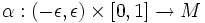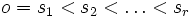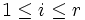# Variation of a path

(Redirected from Variation)

## Definition

Let$M$ be a differential manifold and$\omega$ a path in$M$. A variation of$\omega$ is a map$\alpha: (-\epsilon, \epsilon) \times [0,1] \to M$ such that$\alpha(0,t) = \omega(t) \forall t$.

For a given

## Properties

### Smooth variation

Further information: Smooth variation

A smooth variation is a variation for which the map$\alpha$ is smooth from the product manifold to$M$. Note that only a smooth path can admit a smooth variation,

### Piecewise smooth variation

Further information: Piecewise smooth variation

A piecewise smooth variation is a variation for which we can find$o = s_1 < s_2 < \ldots < s_r$ such that$\alpha$ restricted to$(-\epsilon,\epsilon) \times [s_{i-1},s_i]$ is smooth for$1 \le i \le r$. Note that only a piecewise smooth path can admit a piecewise smooth variation.